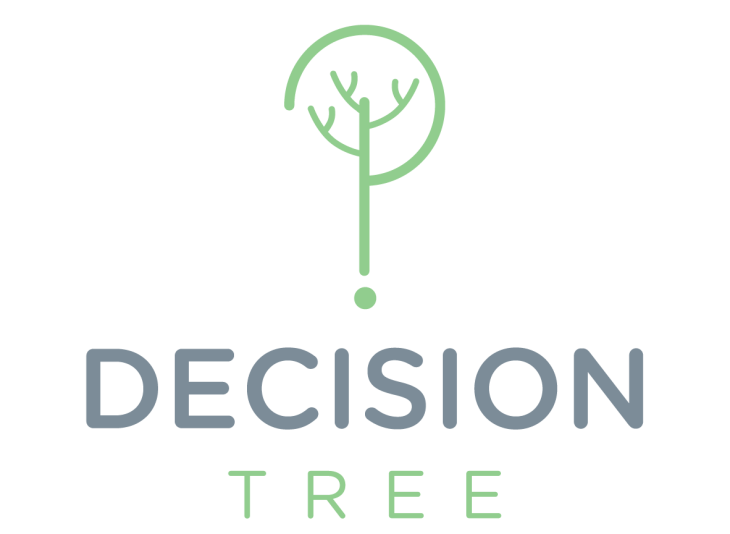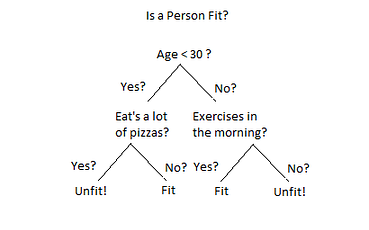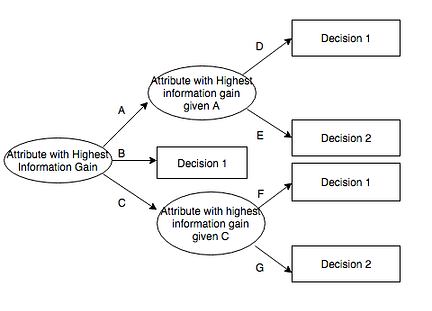By- Vineet Maheshwari
To infinity and beyond.

0 likes followers ViewsVineet Maheshwari

Followers
Views
0 Likes

# Decision TreesTrees have influenced a wide area of Machine Learning. Decision Trees or D-Tress are a form of non-parametric supervised learning method, used for classification and regression. It predict various outcomes by making some decisions. In these trees, features are represented by a node and a decision is represented by a branch or a link. The topmost node is called root node or the parent node, or we can say that at that point we get to know about the problem properly.Now for example, here we can say that the first question, Age < 30 ? This question is the root node or parent question. Now it is a point where we have to take decision. So the link between these two answers with the parent is the decision being made. Till now we have developed a basic understanding about decision tress. Now we will learn about various type of decision trees there applications and many more things.

Types of Decision Trees :

There are many types of decision trees that can be used, but broadly it is classified into 2 types :

1. Classification Trees : In this type, analysis takes place when the predicted outcome belongs is the class to which our original data belongs. For example, outcome of crossing a road as risky or not.
2. Regression Tree : Analysis takes place when the predicted outcome can be considered a real number. For example number of members in a team.source : wikipedia

3. ID3 : Iterative Dichotomiser is an algorithm that generates a decision tree from the data. It uses a top-down approach and performs a greedy search through the data set. Each attribute is tested at each tree node to get the best attribute for classification. Therefore at last, the attribute with the highest information gain is used at particular tree node. It only accepts categorical attributes.

4. C 4.5 : It is an extension of ID3 algorithm. It creates decision trees that can be used for classification, therefore it is also referred as Statistical Classifier. It is better than ID3 because it deals with continuous and discrete attributes, it also works well with missing values. C 5.0 is a successor of C4.5 because it is much faster than it and more memory efficient.

5. CART : Classification and Regression Trees, they are used for both the purpose classification and regression. CART uses binary splitting of attributes for the formation of decision trees using classification. And the splitting attributes are being selected by Gini Index. It is also used for regression analysis. It also supports both continuous and nominal attribute data.

So we talked about splitting of data, now we will look into various attribute measures that Decision Trees use for splitting of attributes.

Attribute Selection Measures :

1. Gini Index : Gini index measures the impurity, a data partition or set of tuples as :

Gini(D) = 1 - Σ(p^2)

Here p is the probability, that a tuple in D belongs to class Ci.

And this is estimated by Ci/D. The sum is computed over m classes.

2. Entropy : It is a measure of uncertainty associated with a random variable. And is directly proportional to uncertainty of randomness. The value of entropy ranges from 0–1.

Entropy(D) = Σ - pi(log2(pi))

Here also p is probability. And calculation is same to that of Gini Index.

3. Information Gain : ID3 uses this technique for attribute selection measure. It is the difference between the original information requirement (based on the original proportion of class) and the new requirement.

Gain(D,A) = Entropy(D) - Σ(((Dj)/D)*Entropy(Dj))

D is the given data partition

A is attribute, an attribute can have V distinct values.

So these were the techniques, now we will look into the applications of Decision Trees.

1. It stands as an effective tool for mineral classification.
2. It is very useful in industries for producing quality control system.
3. It is an important technique that is widely used in medical research and science.
4. It is widely used in the field of E-commerce, helps to generate online catalog, which is very important for the success of E-commerce.
5. Used for visualization of probabilistic business models, used in Customer Relationship Management and used for credit scoring for credit card users.